New Analytical Formulae to Calibrate Well-type Scintillation Detectors Efficiency | OMICS International
Journal of Physical Mathematics

# New Analytical Formulae to Calibrate Well-type Scintillation Detectors Efficiency

Abbas MI1*, Ibrahim OA2, Ibrahim T1,2 and Sakr M1,2

1Department of Physics, Faculty of Science, Alexandria University, Egypt

2Department of Physics, Faculty of Science, Beirut Arab University, Lebanon

*Corresponding Author:
Abbas MI
Department of Physics, Faculty of Science
Alexandria University, Egypt
Tel: 20 3 5921675
E-mail: [email protected]

Received Date: February 03, 2016; Accepted Date: April 14, 2016; Published Date: April 20, 2016

Citation: Abbas MI, Ibrahim OA, Ibrahim T, Sakr M (2016) New Analytical Formulae to Calibrate Well-type Scintillation Detectors Efficiency. J Phys Math 7:164. doi:10.4172/2090-0902.1000164

Copyright: © 2016 Abbas MI, et al. This is an open-access article distributed under the terms of the Creative Commons Attribution License, which permits unrestricted use, distribution, and reproduction in any medium, provided the original author and source are credited.

Visit for more related articles at Journal of Physical Mathematics

#### Abstract

Direct Mathematical calculations for the absolute efficiency of well-type gamma ray scintillation crystals are described. Calculated detection efficiencies are drawn for two commonly used well crystals. Comparisons are made between present works and published Monte Carlo values. The present approach proved quite success in predicting the efficiency of well type detectors providing only the geometry and materials of the system formed of source, detector and shielding as well as the energy of the emitted photons.

#### Keywords

New mathematical method; Gamma-spectroscopy; Absolute efficiency; Well-type detector

#### Introduction

Direct mathematical method to calculate the efficiency of radioactive systems was first proposed by Selim and Abbas and then it was used by several authors [1-12]. The comparison between the calculated values of efficiencies and experimental ones provided in these papers shows that the error is very small. This method proves its accuracy and success for many detector geometries; like: coaxial detectors [1-4], parallelepiped detectors  and borehole detectors ; and types like: semiconductors [4-7] and scintillators [1-3] Besides, the direct mathematical method is built on simple mathematical approach and it does not need long computer programs nor previous experimental calculation of the efficiency of standard source like other Monte Carlo method [13-16]. In this work we used direct mathematical method to predict the efficiency of two well type detectors, in detecting the gamma photons produced by sources placed in their well cavities, over wide range of photon energy for seven different source geometries and positions including point sources with very small dimensions and cylindrical sources. These detectors are scintillators and their active material is Sodium Iodide (NaI). Sodium Iodide is widely used in detecting of gamma photons nowadays because of the high efficiency of these crystals, relatively low cost and availability in different shapes and sizes. In our calculation, the effect of beam attenuation caused by well’s walls and bottom is included as well as self-attenuation caused by the material of cylindrical sources.

For each source geometry, a comparison between our calculated value of efficiencies using this approach and reference values obtained from Pomme et al.  is given. This comparison shows that our values were in good agreement with Pomme et al. , proving the success of our approach in predicting the efficiencies of these detectors over wide range of photon energy.

The arrangement of this paper is as follows: providing a general explanation of the direct mathematical method in section 2, geometric description of well type detector is given in section 3. Detailed explanation of the mathematical perspective used is done. Comparison between calculated and reference values of efficiency is done in seven independent figures. Finally the conclusion is presented.

#### Direct Mathematical Method

This method is theoretical based on the following fundamentals: When a photon with certain energy strikes a detector without absorbing walls or windows, the probability for it to interact with this detector and depose energy to be recorded is:

f =1− e−μ.d        (1)

Where, μ represents the total attenuation coefficient of the detector active volume, and d, is the photon path length traveled through the detector active volume. The factor determining the beam attenuation due the source container and the detector end cap materials is called the attenuation factor, Fatt. So the probability is rewritten as:

fj =Fatt(1− e−μ.d)           (2)

The attenuation factor Fatt, is expressed as: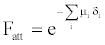(3)

Where, μi is the attenuation coefficient of the ith absorber for a gamma photon with energy Eγ and δi is the gamma photon path length through the ith absorber.

#### Geometric Description of Well Type Detector

The detector, we calculated its efficiency, is a well type NaI detector of cylindrical shape. This detector is illustrated in Figure 1. The total height of this detector is marked as “T” in the mentioned figure and its total radius “R”. This detector contains a coaxial cavity (well) of cylindrical shape also that has a radius r and a height D as shown in Figure 1. A small radioactive source can be placed in the well coaxial cavity. The source might be a small cylinder that can fit in the cavity or an extremely small source (point source). This source contains usually radioactive nuclei that undergo beta or alpha decay, and then emit gamma photons.

Figure 1: Three dimensional sketches and a vertical section view for a well type detector.

#### Mathematical Perspective

Path-lengths

There are five different path lengths “faiths” (d1, d2, d3, d4 and d5) can be followed by a photon in our system depending on where the photon enters the active material and leaves it, the photon can enter either from well’s bottom or the well’s side and leaves either from detector bottom, upper face or outer side.

If the photon enters the active material from the bottom and leaves from the bottom: Just by making projection on the axis of the detector, one can derive the function of path length to be: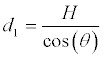(4)

If the photon enters the active material from well’s side and leaves from detector’s bottom: By making projections on a vertical and horizontal planes (Figure 2). We can derive the path length function of the distance covered in the active material (NaI) in terms of the detector and source dimensions shown in Figure 1, as well as the polar and azimuth angle. As shown in Figure 2. If the photon enters from the side of the well cavity and leaves from the detector’s bottom, the distance it covered inside the detecting material (NaI) is labeled by CD (d) in the figure, the distance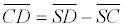where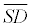can be taken from the vertical view (left) to be z/cos(θ), and SC is simply S’C/sin(θ). The horizontal view to the right of the same figure. Figure 2 shows the triangle OXC right at X, the simple geometry of OXC gives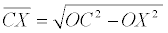and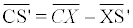; but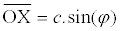and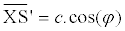and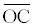= r. So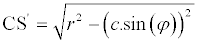the path length covered by a photon entering from the well’s side and leaving from the detector’s bottom will be: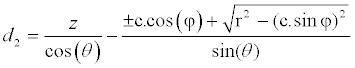(5)

Figure 2: Three dimensional schematic diagram of a well type detector (left) and to a horizontal view plane (right) to illustrate the path length function d2.

To discuss the “±” sign in the equation of path length d2, we consider the projection on a horizontal plane containing the point where the photon enters the active material shown in Figure 3, the photon can enter the active material from the nearer arc shown as dashed arc in the figure or from the farther arc shown as solid arc. If it enters from the nearer arc (left part of Figure 3), the distance will be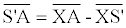and thus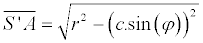. If the photon enters from the farther arc (right part of the figure), the distance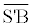in the figure is done by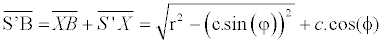.

If the photon enters the active material from well’s bottom and leaves from the outer side: As shown in Figure 4, if the photon enters from the well’s bottom and leaves from detector’s outer side the distance covered inside the active material is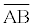where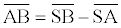SA is clearly f/cos(θ) and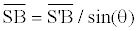. To derive S’B with respect to R, and φ, the horizontal view to the right is done,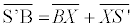here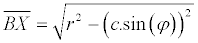and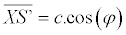Figure 3: Horizontal View made to discuss the “±” sign in the equation of the path length: d2.

Figure 4: Three dimensional sketch and horizontal view made to derive the path length function: d3.

So,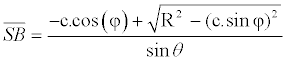and the path length function “d3” in this case will be: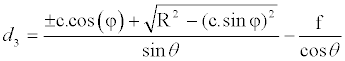(6)

If the photon enters the active material from the well’s wall and leaves from the outer wall: If the photon enters from the well’s wall, the total distance it covers inside the well cavity is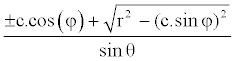(derived previously to be used in equation 5). While if it leaves from the detector’s outer side, the distance it covers in the entire system (active material + well) is(derived previously to be used in equation 6). So the distance covered in the active material will be: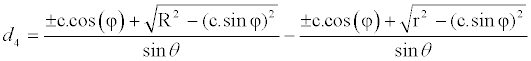(7)

If the photon enters the active material from the inner side and leaves from the upper surface: To derive the path length function d5; Figure 5 shows three dimensional diagram for a photon entering from well’s wall and leaving from detector’s upper surface the total distance the photon covers in the entire system(well and active material) labeled as SF in the figure is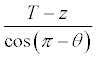while the distance it covers in the well is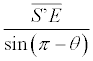S’E can be derived using the horizontal view in the same figure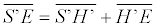. So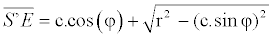Figure 5: Three dimensional diagrams (left) and a projection to the plane containing the point E where the photon enters the active material (NaI).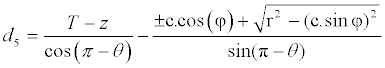(8)

Note: for an axial point source all items multiplied by “c” in the previous equations tend to zero.

Total efficiency of an axial point source

By definition, the efficiency of a source - detector system is the number of photons detected divided by the number of photons emitted. So, taking the total solid angle to be 4π, and using equation (2) we obtain: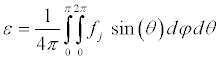(9)

Where,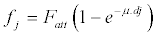(j → 1 – 5) dj can be one of the five functions described before in section 4.1. So to develop equation 9 we just want to replace dj (j → 1 – 5) by its function and we integrate over the entire solid angle taking into account that it might depend on both φ and θ. But in the case of an axial point source, there is symmetry on the azimuth angle φ. We have to distinguish between two cases

If the axial point source is located at a height,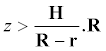(Figure 6, right):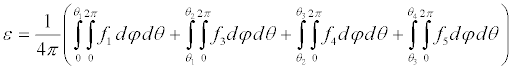(10)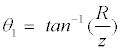;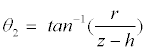;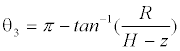;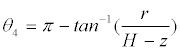(11)

If the axial point source is located at a height,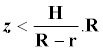(refer to Figure 6, left): applying the equation (2) gives: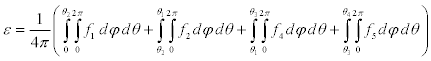(12)

Total efficiency of a non-axial point source

For a non-axial point source one can calculate the total efficiency by applying equation 9, but first we have to take references for the azimuth angle “φ” as well as for the polar angle “θ”. Since each point should belong to certain diameter we take the diameter containing the point-source as the reference of azimuthal angle “φ=0” in order to obtain certain symmetry in the Figure 7a. As well as we take the line parallel to the detector axis passing by the point source is the reference of polar angle “θ=0”. The plane passing by the point source and containing the axis of the detector as well as the horizontal line having φ=0; can be considered as plane of reference in our case because it cuts the detector into two identical parts. We divide the detector into four zones as indicated in Figure 7b. The aim of this technique is to make the equations easier. The two regions to the right are considered closer to the source, so the signs (±) in the equations (6 – 8) will turn to (–). Figure 7a shows a 3D- drawing showing the references of the polar and azimuthal angles.

Figure 6: Vertical section view of the detector with an axial point source in two cases: Case 1 shows a lower point source below with z H/(R-r).R (to the right). Note: z, R, H and r were defined in Figure 1.

Figure 7a: Shows a 3D- drawing showing the references of the polar and azimuthal angles.

Zone A, the point is closer to the detector axis and 0 < θ < π/2: To derive the mathematical equations, we will know first the photon path lengths allowed in this zone. Here the photon can enter either from the well cavity bottom or side and leave either from the outer side or bottom of detector. Thus, four path lengths will be allowed in this case which are: d1, d2, d3 and d4 (Tables 1 and 2). In this section we will study the different faiths when the photon enters the detector, as well as the different faiths when this photon leaves the detector, then we combine them to find different faiths of photon path length in the detector active volume. We will do the same strategy for the other zones. First we will study how the photon leaves the detector, we will make a projection on a horizontal plane containing the bottom of the detector so, as illustrated in Figure 8, we obtain:

The proton enters from The proton leaves from θ < θ1bottom θ1< θ < θ2
f<faside
θ1< θ < θ2
f >fabottom
θ > θ2side
θ < θ3the well cavity bottom d1 d3 d1 d3
θ3< θ < θ4 and f <fa' the well cavity side d2 d4 d2 d4
θ3< θ < θ4 and f >fa' the well cavity bottom d1 d3 d1 d3
θ  > θ4 the well cavity side d2 d4 d2 d4

Table 1: The path length functions allowed for a photon striking part A of the detector. So, in general the photon path length can follow one of the path lengths d1, d2, d3 and d4. Table 1 summarizes the faiths that can be followed by the photon.

The proton enters
from
The proton leaves from θ < θ2   bottom θ2< θ < θ6
f <fb bottom
θ2< θ < θ6
f >fb side
θ > θ2
side
θ < θ4 the well cavity bottom d1 d1 d3 d3
θ4< θ < θ5 and f <fa' the well cavity bottom d1 d1 d3 d3
θ4< θ < θ5 and f >fa' the well cavity side d2 d2 d4 d4
θ5<θ the well cavity side d2 d2 d4 d4

Table 2: The path length Functions allowed for a photon striking part B of the detector. So, in general the photon path length can follow one of the path lengths d1, d2, d3 and d4. Table 2 summarizes the faiths that can be followed by the photon.

Figure 8: Projection on the horizontal plane containing the well’s bottom (left) to show the projection of angles θ3 and θ4 and azimuth angle φa’, and a projection on the detector’s bottom (right) to show the projection of angles θ1 and θ2 and azimuth angle φa. Figure

• For θ < θ1; the photon must leave from the bottom.

• For θ1< θ < θ2; the photon can leave from the side up to certain azimuth angle φa, while it leaves from the bottom when φ > φa.

• For θ > θ2; the photon must leave from the side.

Also, Figure 8 allows us to derive the equations of θ1 and θ2: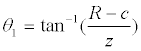(13)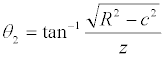(14)

Also from Figure 8, it is easy to derive the equation of φa by applying the cosine law in triangle SOC; we have: (SO)2 + (SC)2 + 2 (SO).(SC). cos(φa) = (OC)2, which turns after substitution into: c2 +z2.tan2(θ) + 2.c.z.tan(θ).cos(φa) = R2, thus φa can be done by: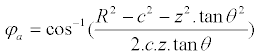(15)

Now to study how the photon can enter the detector we will make projection on the plane containing the bottom of the well, the projection of the polar angle “θ” on a horizontal plane is a circle with radius [f x tan(θ)], where f designates the height of the source above the plane of projection. As shown in Figure 8; for θ < θ3 the photon must enter from the bottom of well. But for θ3 < θ < θ4, the photon can enter from the side if it has an azimuthal angle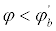For θ > θ4; the photon must enter from the side. Also from Figure 8 we can derive easily the functions of θ3 and θ4, it is clear: sin(θ4) = (√(r2-c2)/ hypotenuse) while cos(θ4) = (f/ hypotenuse), so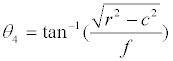(16)

and, in a similar manner:(17)

In addition we can use Figure 8 to derive the equation of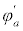by applying the cosine law in triangle S’O’C’; we have: (S’O’)2 + (O’C’)2 + 2.(S’O’).(O’C’).cos () = (S’C’)2, which turns after substitution into: c2 +f 2.tan2(θ) + 2.c.f.tan(θ).cos() = r2, thuscan be done by: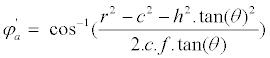(18)

Zone B, the further zone with 0 < θ < π/2: Just like we studied in zone A, the path length functions followed by the photon in the detector if it strikes zone B will depend on two things only: how it enters and how it leaves the detector material. The photon can enter from either the bottom or the side of the well cavity; we should study the different faiths depending on polar and azimuth angles. Starting by studying the faiths of entrance, we have to make projection on a plane containing the bottom of well cavity, thus according to Figure 9 (left), we obtain:

Figure 9: Projection on two horizontal planes (the plane containing the well’s bottom to the left and the one containing the detector’s bottom to the right) to discuss the path length functions followed by a photon crossing zone B of the detector.

For θ < θ4; the photon must enter from the bottom, whatever the azimuth angle is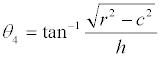where (as identified before in equation 16)

For θ4 < θ < θ4; the photon enters the detector from the bottom if it has a small azimuthal angleor from the side if it has a large azimuthal anglewhere: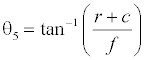(19)

For θ > θ5; the photon must enter from well’s side. While, if we study the faiths the photon will leave the detector according to the polar and azimuthal. For θ < θ2; the photon must leave from the bottom whatever the azimuth angle is; where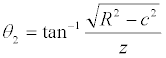(as identified before in equation 14);

For θ2 < θ < θ6; the photon might leave the detector from the bottom if it has a small azimuthal angle (φ < φb) or from the side if it has a large azimuthal angle (φ > φb), where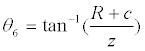(20)

• φb is done in equation 21

• For θ > θ6; the photon must leave from the side.

Here, because we are dealing with the bigger part of the detector, so the sign (±) in the equations (5 – 7) of d2, d3 and d4 will turn to (+). By looking at Figure 9, we take the triangle BSO and applying the cosine law in simple mathematics. We have:

(OB)2 = (OS)2 + (SB)2 +2.(SO).(SB).cos(φb), but (OS) = c, (OB) = r and (SB) = h tan(θ), so we obtain: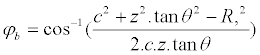(21)

Now, it is easy to derive the equation of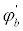, just by applying the same method and taking the triangle S’O’B’, we obtain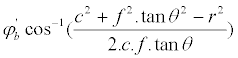(22)

Zone C: The part of the detector close to the source with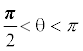: The particle must enter from the side, while it can leave either from the upper face or the outer side so two path lengths might take place here: d4 and d5. This depends on the polar and azimuth angles certainly, to study the path followed by the photon according to these angles we can make projection on a horizontal plane but now the horizontal plane will be the one containing the upper face of the detector. Here, unlike zones A and B, we need projection on one single plane. The projection is shown in Figure 10. By taking the triangle OSY; OS = c, OY = r and SY = (T-z).tan(π-θ), we can derive the function of φc and by taking the triangle OSX we can derive the equation of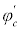Figure 10: Projection to a horizontal plane containing the surface of detector the right figure can be used to determine the equations of φc and φc’.

If θ < (π-θ7), the photon must leave from the side; thus it is going to follow the path length d4, where: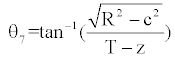(23)

If (π-θ7) < θ < (π-θ8), the photon can leave either from upper face if its azimuthal angle is less then certain value we can call it φc or from the side if it has an azimuthal angle φ > φc, where: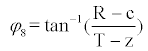(24)

Also, φc can be deduced from Figure 10, and in a way similar to φa: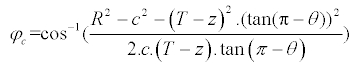(25)

If (π-θ8) < θ < (π-θ9), the photon must leave from the upper face, where: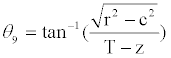(26)

If (π-θ9) < θ < (π-θ10), the photon can either leave from the upper face if its azimuthal angle is less than certain value we call itor escape without hitting the active material of the detector for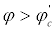where: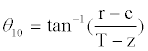(27)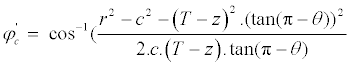(28)

If θ > (π-θ10), the photon leaves the detector without hitting its active material.

Zone D: The farther part with θ > π/2: Also for the farther part with polar angle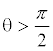; the photon must enter from the side while it can leave either from side or upper face. We should study whether the photon will leave from the upper face or from the side by making a projection on the plane containing the upper face of detector just like we did in the previous part. The projection is shown in Figure 11.

• If θ < (π- θ11), the photon must leave from the side and the only path way followed is d4, where: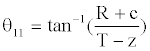(29)

• If (π-θ11) < θ < (π-θ7), the photon can leave either from side or upper face depending on the azimuthal angle. It leaves from upper face for azimuthal angle φ < φd and it leaves from side if φ>φd.

• If (π-θ7) < θ < (π-θ12), the photon must leave from the upper face whatever the azimuthal angle was, where: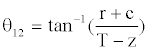(30)

If (π-θ12) < θ < (π- θ9), the photon can escape without striking the material of detector for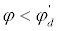or strike it entering from the side and leaving from the face if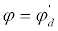• For θ > (π-θ9), the photon cannot strike the detector.

Also, Figure 11 helps to derive the equations of the new azimuthal angles: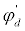and ∅d in a manner very similar to ∅c and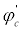.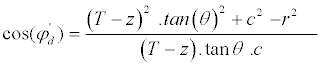(31)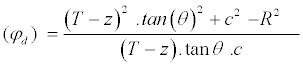(32)

Thus the efficiency of the detector for a non-axial point source will be done by: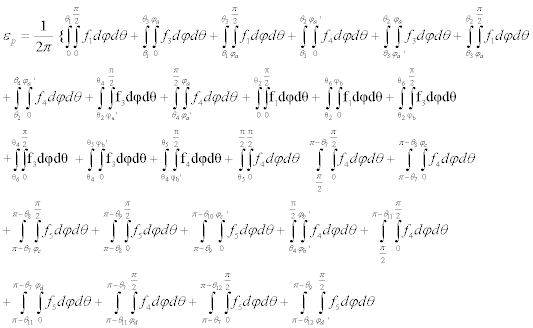(33)

Where, f is as identified before in equation 2. The previous equation can be used for θ3 < θ2 and θ6< θ4.

#### Absolute Efficiency for Disk and Cylindrical Sources

The efficiency of a circular disk source of radius S can be done by: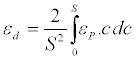(34)

Where εP is the efficiency of a non-axial point source, as identified before in equation (33).In the case of cylindrical sources, we can calculate the efficiency by making an integration of εP over dc and dh and taking into consideration the efficiencies of many point sources on the same shell are equals. So for a cylinder of radius S and height H we have: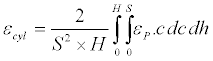(35)

#### Results and Comparisons

In this study we used two well type detectors , (1) IRMM is used in Figures 12-17 and (2) CIEMAT is used in Figure 18. The dimensions of the two detectors are given in Table 3. The following figures illustrate the comparison between the theoretical calculated values (present work) and the published Monte Carlo values for different sources. The percentage deviation is given by: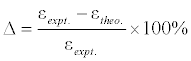(36)

Figure 12: Shows the present work and Monte Carlo  values for an axial point source positioned at height z=8.55 cm, from the well bottom of detector IRMM, without source-walls.

Figure 13: Shows the present work and Monte Carlo  values for a nonaxial point source positioned at height z=5.66 cm, from the well bottom of detector IRMM, without source-walls.

Figure 14: Shows the present work and Monte Carlo  values an eccentric cylindrical source, with 2 cm radius and 4 cm height, located at z=6.05 cm, from the well bottom of detector IRMM, within a thick Acrylic container with walls (bottom and surface) of 0.1 cm thickness.

Figure 15: Shows the present work and Monte Carlo  values for a coaxial cylindrical source, with 2 cm radius and 4 cm height, and its bottom is located at a height z=6.05 cm, from the well bottom of detector IRMM, within a thick Acrylic container with walls (bottom and surface) of 0.1 cm thickness.

Figure 16: Shows the present work and Monte Carlo  values for an axial point source located at a height z=5.55 cm, from the well bottom of detector IRMM, within a thick Acrylic container with walls (bottom and surface) of 2.5 cm thickness.

Figure 17: Shows the present work and Monte Carlo  values for an axial point source located at a height z=7.85 cm, from the well bottom of detector IRMM, within an Aluminum container with walls of 1 cm thickness and its bottom and surface of thickness 0.2 cm.

Figure 18: Shows the present work and Monte Carlo  values for a coaxial disk source, with radius=0.15 cm, located at a height z=2.61 cm, from the well bottom of detector CIEMAT, within a very thin Acrylic container.

where,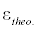and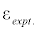are the theoretical (present work) and the Monte Carlo efficiencies, respectively [17-21]

#### Conclusion

The present approach offers straightforward mathematical equations to calibrate well-type NaI(Tl) scintillation detector over a wide energy range. This approach is based on simple mathematical integrals and computer programs to calculate the detection efficiency. It does not need neither optimization of the detector parameters or previous knowledge of a standard source values. The comparison between calculated and reference values shows that the difference is very small (never escaped 8% and reduced to less than 2% in most cases).

#### References

Select your language of interest to view the total content in your interested language

### Article Usage

• Total views: 10521
• [From(publication date):
June-2016 - Jan 18, 2020]
• Breakdown by view type
• HTML page views : 10221Can't read the image? click here to refresh